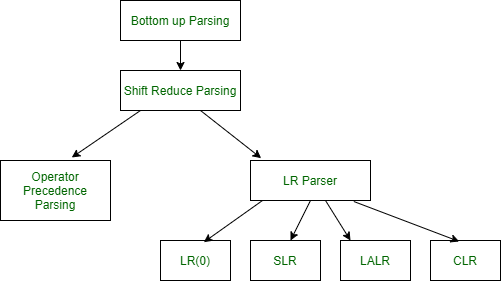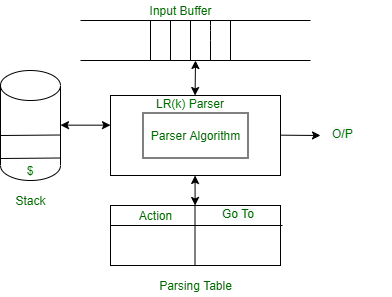Open in App
Not now

# LR Parser

• Difficulty Level : Medium
• Last Updated : 31 Mar, 2021

In this article, we will discuss LR parser, and it’s overview and then will discuss the algorithm. Also, we will discuss the parsing table and LR parser working diagram. Let’s discuss it one by one.

LR parser :
LR parser is a bottom-up parser for context-free grammar that is very generally used by computer programming language compiler and other associated tools. LR parser reads their input from left to right and produces a right-most derivation. It is called a Bottom-up parser because it attempts to reduce the top-level grammar productions by building up from the leaves. LR parsers are the most powerful parser of all deterministic parsers in practice.Description of LR parser :
The term parser LR(k) parser, here the L refers to the left-to-right scanning, R refers to the rightmost derivation in reverse and k refers to the number of unconsumed “look ahead” input symbols that are used in making parser decisions. Typically, k is 1 and is often omitted. A context-free grammar is called LR (k) if the LR (k) parser exists for it. This first reduces the sequence of tokens to the left. But when we read from above, the derivation order first extends to non-terminal.

1. The stack is empty, and we are looking to reduce the rule by S’→S\$.
2. Using a “.” in the rule represents how many of the rules are already on the stack.
3. A dotted item, or simply, the item is a production rule with a dot indicating how much RHS has so far been recognized. Closing an item is used to see what production rules can be used to expand the current structure. It is calculated as follows:

Rules for LR parser :
The rules of LR parser as follows.

1. The first item from the given grammar rules adds itself as the first closed set.
2. If an object is present in the closure of the form A→ α. β. γ, where the next symbol after the symbol is non-terminal, add the symbol’s production rules where the dot precedes the first item.
3. Repeat steps (B) and (C) for new items added under (B).

LR parser algorithm :
LR Parsing algorithm is the same for all the parser, but the parsing table is different for each parser. It consists following components as follows.

1. Input Buffer –
It contains the given string, and it ends with a \$ symbol.

2. Stack –
The combination of state symbol and current input symbol is used to refer to the parsing table in order to take the parsing decisions.

Parsing Table
Parsing table is divided into two parts- Action table and Go-To table. The action table gives a grammar rule to implement the given current state and current terminal in the input stream. There are four cases used in action table as follows.

1. Shift Action- In shift action the present terminal is removed from the input stream and the state n is pushed onto the stack, and it becomes the new present state.
2. Reduce Action- The number m is written to the output stream.
3. The symbol m mentioned in the left-hand side of rule m says that state is removed from the stack.
4. The symbol m mentioned in the left-hand side of rule m says that a new state is looked up in the goto table and made the new current state by pushing it onto the stack.
```An accept - the string is accepted
No action - a syntax error is reported```

Note –
The go-to table indicates which state should proceed.

LR parser diagram :My Personal Notes arrow_drop_up